## Exercises

1. Extend the vertical decomposition algorithm to correctly handle the case in which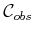has two or more points that lie on the same vertical line. This includes the case of vertical segments. Random perturbations are not allowed.
2. Fully describe and prove the correctness of the bitangent computation method shown in Figure 6.14, which avoids trigonometric functions. Make certain that all types of bitangents (in general position) are considered.
3. Develop an algorithm that uses the plane-sweep principle to efficiently compute a representation of the union of two nonconvex polygons.
4. Extend the vertical cell decomposition algorithm of Section 6.2.2 to work for obstacle boundaries that are described as chains of circular arcs and line segments.
5. Extend the shortest-path roadmap algorithm of Section 6.2.4 to work for obstacle boundaries that are described as chains of circular arcs and line segments.
6. Derive the equation for the Conchoid of Nicomedes, shown in Figure 6.24, for the case of a line-segment robot contacting an obstacle vertex and edge simultaneously.
7. Propose a resolution-complete algorithm for motion planning of the line-segment robot in a polygonal obstacle region. The algorithm should compute exact C-space obstacle slices for any fixed orientation,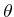; however, the algorithm should use van der Corput sampling over the set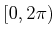of orientations.
8. Determine the result of cylindrical algebraic decomposition for unit spheres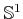,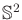,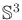,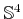,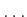. Each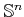is expressed as a unit sphere in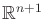. Graphically depict the cases ofand. Also, attempt to develop an expression for the number of cells as a function of.
9. Determine the cylindrical algebraic decomposition for the three intersecting circles shown in Figure 6.43. How many cells are obtained?
10. Using the matrix in (6.28), show that the result of Canny's roadmap for the torus, shown in Figure 6.39, is correct. Use the torus equation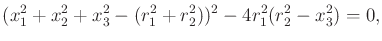(6.40)

in whichis the major circle,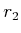is the minor circle, and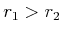.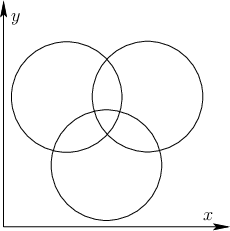11. Propose a vertical decomposition algorithm for a polygonal robot that can translate in the plane and even continuously vary its scale. How would the algorithm be modified to instead work for a robot that can translate or be sheared?
12. Develop a shortest-path roadmap algorithm for a flat torus, defined by identifying opposite edges of a square. Use Euclidean distance but respect the identifications when determining the shortest path. Assume the robot is a point and the obstacles are polygonal.

Implementations

13. Implement the vertical cell decomposition planning algorithm of Section 6.2.2.
14. Implement the maximum-clearance roadmap planning algorithm of Section 6.2.3.
15. Implement a planning algorithm for a point robot that moves in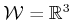among polyhedral obstacles. Use vertical decomposition.
16. Implement an algorithm that performs a cylindrical decomposition of a polygonal obstacle region.
17. Implement an algorithm that computes the cell decomposition of Section 6.3.4 for the line-segment robot.
18. Experiment with cylindrical algebraic decomposition. The project can be greatly facilitated by utilizing existing packages for performing basic operations in computational algebraic geometry.
19. Implement the algorithm proposed in Exercise 7.

Steven M LaValle 2020-08-14